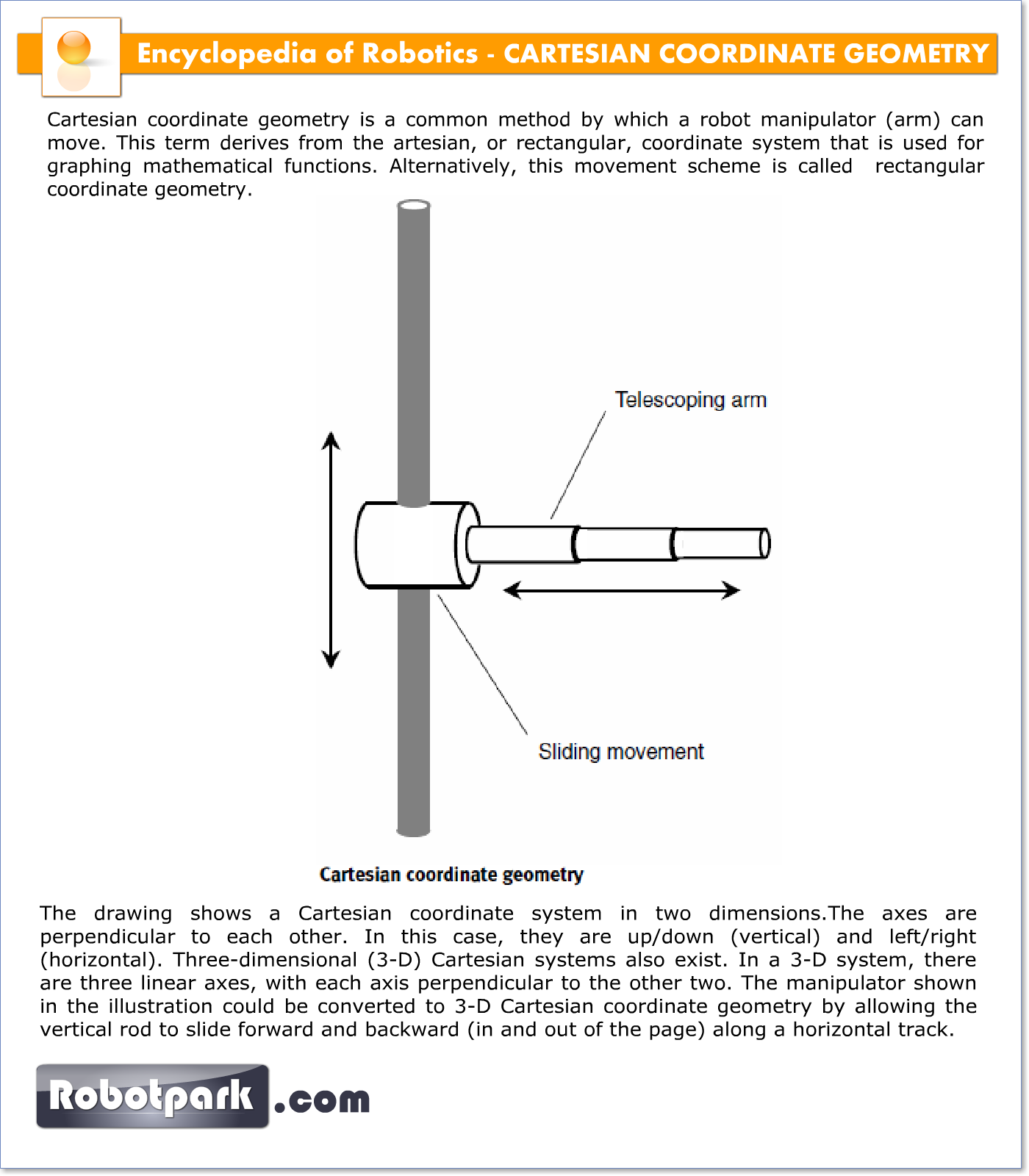# CARTESIAN COORDINATE GEOMETRY – 21027

Cartesian coordinate geometry is a common method by which a robot manipulator (arm) can move. This term derives from the artesian, or rectangular, coordinate system that is used for graphing mathematical functions. Alternatively, this movement scheme is called  rectangular coordinate geometry.The drawing shows a Cartesian coordinate system in two dimensions. The axes are perpendicular to each other. In this case, they are up/down (vertical) and left/right (horizontal). Three-dimensional (3-D) Cartesian systems also exist. In a 3-D system, there are three linear axes, with each axis perpendicular to the other two. The manipulator shown in the illustration could be converted to 3-D Cartesian coordinate geometry by allowing the vertical rod to slide forward and backward (in and out of the page) along a horizontal track.